Checkout JEE MAINS 2022 Question Paper Analysis : Checkout JEE MAINS 2022 Question Paper Analysis :

# JEE Main 2021 March 16 – Shift 2 Chemistry Question Paper with Solutions

JEE Main 2021 March 16th Shift 2 Chemistry question paper along with detailed solutions are provided in this page. Candidates can view and go through the questions and solutions to get a better idea of the overall pattern of JEE Main 2021 March 16th Shift 2 Chemistry paper and also know the correct answers to each of the questions. This will also enable aspirants to understand the type of questions asked in the paper and learn the different methods to solve them. Additionally this will also help them get a deeper understanding of the concepts. Aspirants can download the question paper PDF below (available soon).

### JEE Main 2021 March 16th Shift 2 Chemistry Question Paper

SECTION-A

Question 1. Identify the reagent(s) 'A' and condition(s) for the reaction:1. a. A = HCl; Anhydrous AlCl3
2. b. A = HCl, ZnCl2
3. c. A = Cl2, dark, Anhydrous AlCl3
4. d. A = Cl2; UV Light

Solution: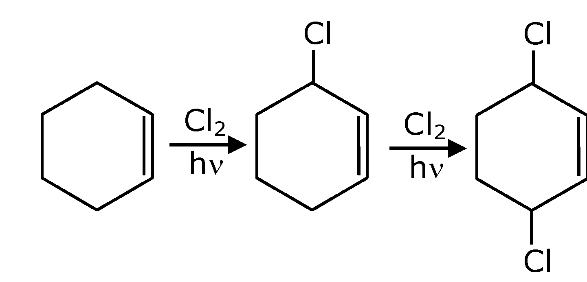Question 2. The INCORRECT statement regarding the structure of C60 is:

1. a. It contains 12 six-membered rings and 24 five-membered rings.
2. b. Each carbon atom forms three sigma bonds.
3. c. The five-membered rings are fused only to six-membered rings.
4. d. The six-membered rings are fused to both six and five-membered rings.

Solution:

It contains 12 five membered rings & 20 six membered rings.

Question 3. Match List-I with List-II:

 List-I List-II Test/Reagents/Observation(s) Species detected (a) Lassaigne's Test (i) Carbon (b) Cu(II) oxide (ii) Sulphur (c) Silver nitrate (iii) N, S, P and halogen (d) The sodium fusion extract gives black precipitate with acetic acid & lead acetate (iv) Halogen Specifically

1. a. (a)-(iii), (b)-(i), (c)-(iv), (d)-(ii)
2. b. (a)-(i), (b)-(iv), (c)-(iii), (d)-(ii)
3. c. (a)-(iii), (b)-(i), (c)-(ii), (d)-(iv)
4. d. (a)-(i), (b)-(ii), (c)-(iv), (d)-(iii)

Solution:

(a)-(iii), (b)-(i), (c)-(iv), (d)-(ii)

Question 4. The structure of X is: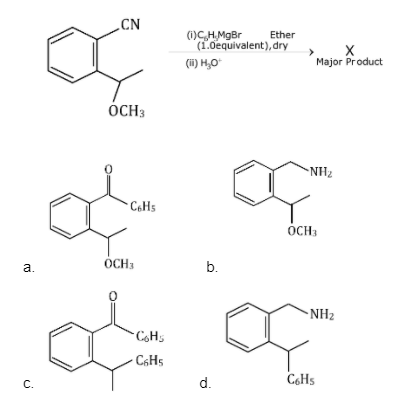Solution: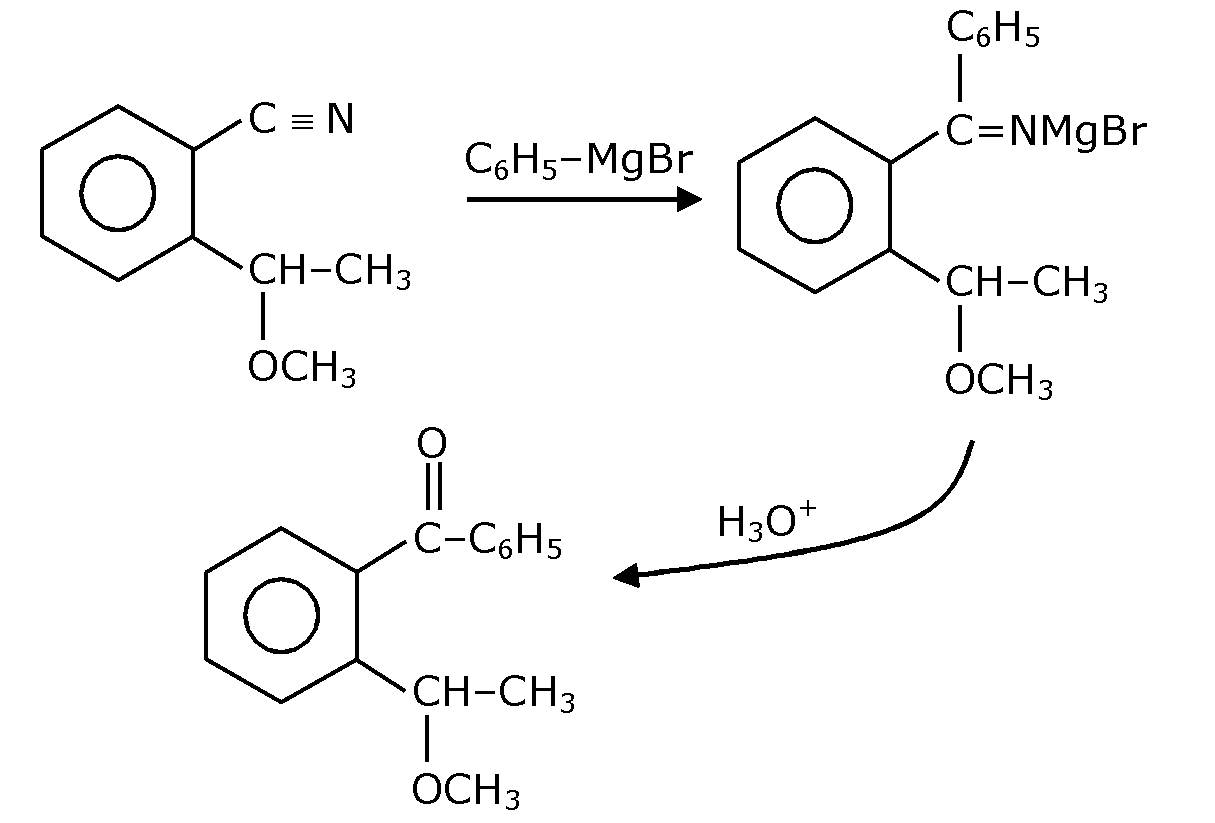Question 5. Ammonolysis of alkyl halides followed by the treatment with NaOH solution can be used to prepare primary, secondary and tertiary amines. The purpose of NaOH in the reaction is:

1. a. to remove basic impurities
2. b. to activate NH3 used in the reaction
3. c. to increase the reactivity of alkyl halide
4. d. to remove acidic impurities

Solution:During the reaction HX (acid) is formed.

Question 6. Arrange the following metal complex/compounds in the increasing order of spin only magnetic moment. Presume all three, high spin systems.

(Atomic numbers Ce = 58, Gd = 64 and Eu = 63)

(1) (NH4)2[Ce(NO3)6]

(2) Gd(NO3)3

(3) Eu(NO3)3

1. a. (1) < (3) < (2)
2. b. (1) < (2) < (3)
3. c. (3) < (1) < (2)
4. d. (2) < (1) < (3)

Solution:

(NH4)2 [Ce(NO3)6] (n = 0) ⇒ µ = 0 B.M

Eu (NO3)3 (n = 6) ⇒ µ = 6.93 B.M

Gd(NO3)3 (n = 7) ⇒ µ = 7.94 B.M

Question 7. Identify the elements X and Y using the ionisation energy values given below:

1. a. X = F; Y = Mg
2. b. X = Mg; Y = F
3. c. X = Na; Y = Mg
4. d. X = Mg; Y = Na

Solution:

2nd I.E of Alkali metals is very high as compared to 1st.

Question 8. The INCORRECT statement below regarding colloidal solutions is:

1. a. A colloidal solution shows colligative properties
2. b. An ordinary filter paper can stop the flow of colloidal particles
3. c. A colloidal solution shows the Brownian motion of colloidal particles
4. d. The flocculating power of Al3+ is more than that of Na

Solution:

Colloidal solutions can pass through ordinary filter paper but cannot pass through special filter colloidal solution coated paper.

Question 9. The characteristics of elements X, Y and Z with atomic numbers, respectively, 33, 53 and 83 are:

1. a. X and Z are non-metals and Y is a metalloid.
2. b. X and Y are metalloids and Z is a metal.
3. c. X, Y and Z are metals.
4. d. X is a metalloid, Y is a non-metal and Z is a metal.

Solution:

Atomic No. Element

1. 33 → As (Metalloid)

2. 53 → I (Non-metal)

3. 83 → Bi (Metal)

Question 10. The exact volumes of 1 M NaOH solution required to neutralise 50 mL of 1 M H3PO3 solution and 100 mL of 2 M H3PO2 solution, respectively, are:

1. a. 100 mL and 50 mL
2. b. 50 mL and 50 mL
3. c. 100 mL and 100 mL
4. d. 100 mL and 200 mL

Solution:

(1) 2NaOH + H3PO3 → Na2HPO3 + 2H2O

100 mmole 50mmole

100 mmole = M × Vml

100 mmole = 1 × Vml

Vml = 100 ml

(2) NaOH + H3PO2 → NaH2PO2 + H2O

200 mmole 200mmole

200 mmole = M × Vml

200 mmole=1 × Vml

Vml = 200 ml

Question 11. Which of the following reduction reaction CANNOT be carried out with coke?

1. a. Fe2O3 → Fe
2. b. ZnO → Zn
3. c. Al2O3 → Al
4. d. Cu2O → Cu

Solution:

Al is extracted by electrolytic reduction of Al2O3.

Question 12. An unsaturated hydrocarbon X on ozonolysis gives A. Compound A when warmed with ammoniacal silver nitrate forms a bright silver mirror along the sides of the test tube. The unsaturated hydrocarbon X is: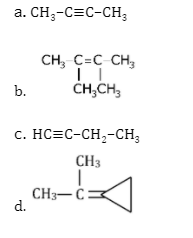Solution:Question 13. Two statements are given below:

Statement-I: Sodium hydride can be used as an oxidising agent.

Statement-II: The lone pair of electrons on nitrogen in pyridine makes it basic.

1. a. Statement I is true but statement II is false
2. b. Both statement I and statement II are false
3. c. Both statement I and statement II are true
4. d. Statement I is false but statement II is true

Solution:

⇒ NaH is used as a reducing agent.

⇒ The l.p. on nitrogen in pyridine makes it basic.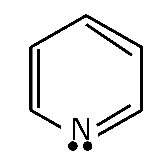Question 14. Which of the following polymer is used in the manufacture of wood laminates?

1. a. Melamine formaldehyde resin
2. b. Cis-poly isoprene
3. c. Phenol and formaldehyde resin
4. d. Urea-formaldehyde resin

Solution:

Melamine formaldehyde resin is used in the manufacture of wood laminates.

Question 15. The correct statements about H2O2 are:

(1) used in the treatment of effluents.

(2) used as both oxidizing and reducing agents.

(3) the two hydroxyl groups lie in the same plane.

(4) miscible with water.

Choose the correct answer from the options given below:

1. a. (1), (3) and (4) only
2. b. (1), (2) and (4) only
3. c. (1), (2), (3) and (4)
4. d. (2), (3) and (4) only

Solution:

(1) Used in the treatment of effluents as it is an oxidizing agent.

(2) In H2O2 oxidation of oxygen is-1. Therefore, it acts both as O.A and R.A.

(3) H2O2 has an open book structure in which both –OH group are not in the same plane

(4) H2O2 is miscible in water due to intermolecular H-Bonding.

Question 16. The greenhouse gas/es is (are)

1. Carbon dioxide

2. Oxygen

3. Water vapour

4. Methane

Choose the most appropriate answer from the options given below:

1. a. (1) and (2) only
2. b. (1), (3) and (4) only
3. c. (1) and (3) only
4. d. (1) only

Solution:

The greenhouse gases are CO2, CH4 & H2O vapour.

Question 17. In the below reaction, the reagent “A” is: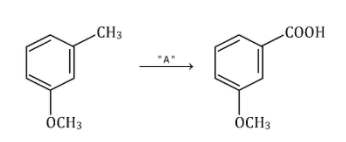1. a. NaBH4, H3O+
2. b. HCl, Zn-Hg
3. c. Alkaline KMnO4, H+
4. d. LiAlH4

Solution: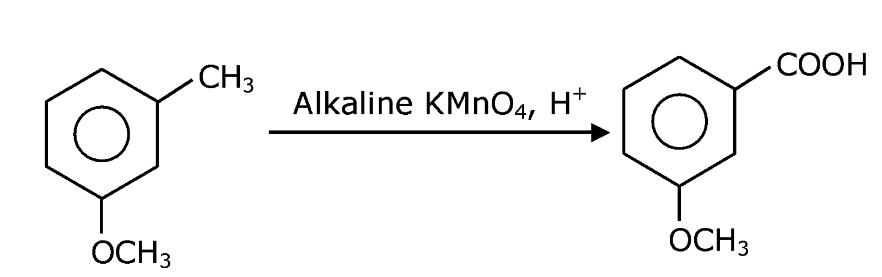Question 18. Which of the following is least basic?

1. a.
$$(CH_{3}CO)_{2}\: \ddot{N}H$$
2. b.
$$(CH_{3}CO)\: \ddot{N}H\: C_{2}H_{5}$$
3. c.
$$(C_{2}H^{5})_{3}\: \ddot{N}$$
4. d.
$$(C_{2}H^{5})_{2}\: \ddot{NH}$$

Solution:Due to higher resonance, lp of N is not available for accepting H+. So, it is least basic.

Question 19. Fex2 and Fey3 are known when x and y are:

1. a. x=Cl, Br, I and y=F, Cl, Br, I
2. b. x=F, Cl, Br, I and y=F, Cl, Br
3. c. x=F, Cl, Br, I and y=F, Cl, Br, I
4. d. x=F, Cl, Br and y =F, Cl, Br, I

Solution:

FeI3, does not form because of I being a very good reducing agent.

Question 20. The secondary structure of protein is stabilized by:

1. a. van der Waals forces
2. b. Peptide bond
3. c. Hydrogen bonding
4. d. Glycosidic bond

Solution:

The secondary structure of protein is stabilized by H-bonding.

Section B

Question 21. At 25°C, 50 g of iron reacts with HCl to form FeCl2. The evolved hydrogen gas expands against a constant pressure of 1 bar. The work done by the gas during this expansion is ________________ J. (Round off to the nearest integer).

[Given: R = 8.14 J mol–1 K–1. Assume, hydrogen is an ideal gas]

[Atomic mass of Fe is 55.85 u]

Solution:

Fe + 2HCl → FeCl2 + H2 (g)

50g

Moles of Fe = 50 / 55.85 mol = Moles of H2

Wirrev = −Pext . ΔV

= − moles of H2 × RT

= − 50 / 55.85 × 8.314 × 298

= − 2218.05 J

Nearest integer = 2218

Question 22. A 5.0 m moldm–3 aqueous solution of KCl has a conductance of 0.55 mS when measured in a cell of cell constant 1.3 cm–1. The molar conductivity of this solution is ___________ mSm2mol1. (Round off to the nearest integer).

Solution:

GKCl = 0.55 mS = 55 × 10–5 s

Cell constant = l/A = 1.3 cm–1

λM = ??

K = G(l/A) = 55 × 10–5 × 1.3 Scm–1

$$\lambda _{M}=\frac{K\times1000}{Molarity} = \frac{55\times1.3 \times10^{-5} \times 1000}{5\times 10^{-3}}$$

λM = 11 × 1.3 × 10 = 11 × 13 = 143 Scm2mol-1

$$\lambda _{M}= \frac{143\times 1000 \times10^{-3}S}{(10^{-2}m)^{-2}} mol^{-1}$$

λM = 143 × 1000 × 10-4 (m.S)m2.mol-1

λM = 14.3 (m.S)m2.mol-1

Nearest integer = 14

Question 23. The number of orbitals with n = 5, m1 = +2 is ___________. (Round off to the nearest integer).

Solution:

For n = 5

l = 0, 1, 2, 3, 4

l = 2 → m = –2, –1, 0, +1, +2

l = 3 → m = –3, –2, –1, 0, +1, +2, +3

l = 4 → m = –4, –3, –2, –1, 0, +1, +2, +3, +4

Total no. of orbitals = 3

Question 24. A and B decompose via first-order kinetics with half-lives 54.0 min and 18.0 min respectively. Starting from an equimolar non-reactive mixture of A and B, the time taken for the concentration of A to become 16 times that of B is __________ min. (Round off to the nearest integer).

Solution:

$$A\overset{1st Order}{\rightarrow}t_{\frac{1}{2}}(A) = 54$$
$$B\overset{1st Order}{\rightarrow}t_{\frac{1}{2}}(B) = 18$$

A0 = B0 = N0

$$A_{t} = \frac{A_{o}}{\frac{2t}{54}}$$
$$B_{t} = \frac{B_{o}}{\frac{2t}{18}}$$

⇒ At = 16.Bt

$$\frac{A_{o}}{\frac{2t}{54}} = 16\times \frac{B_{o}}{\frac{2t}{18}}$$

⇒ 2t/18 – t/54 = 16

⇒ 22t/54 = 16 = 24

⇒ 2t / 54= 4

⇒ t = 108 min

Question 25. [Ti(H2O)6]3+ absorbs light of wavelength 498 nm during a d–d transition. The octahedral splitting energy for the above complex is ___________ × 10–19 J. (Round off to the nearest integer). h = 6.626 × 10–34 Js; c = 3 × 108 ms–1.

Solution:

$$\Delta _{o} = \frac{hc}{I_{abs}} = \frac{6.626\times10^{-34} \times3 \times10^{8} }{498\times10^{-9} }$$

$$= \frac{6.626\times3}{498}\times 10^{-17} = 3.99 \times 10^{-19}$$

Nearest integer = 4 × 10-19 J

Question 26. Sulphurous acid (H2SO3) has Ka1 = 1.7 × 10–2 and Ka2 = 6.4 × 10–8. The pH of 0.588 M H2SO3 is _________. (Round off to the nearest integer)

Solution: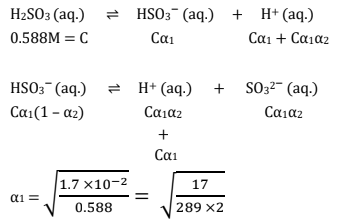Therefore,

α1 << 1 ⇒ (1 – α1) ≃ 1

Hence, α2 << 1 ⇒ (1 – α2) ≃ 1

∴ [H+] = Cα1

=

$$\sqrt{K_{a1}\times c} = \sqrt{17\times10^{-3} \times0.588}$$

= 0.099

pH = -log100.099=1

Nearest integer = 1

Question 27. In Duma's method of estimation of nitrogen, 0.1840 g of an organic compound gave 30 mL of nitrogen collected at 287 K and 758 mm of Hg pressure. The percentage composition of nitrogen in the compound is __________. (Round off to the nearest integer). [Given: Aqueous tension at 287 K = 14 mm of Hg]

Solution:

Moles of N2 =

$$\frac{758-14}{760}\times \frac{30\times 10^{-3}}{0.0821\times 287}$$

= 1.246 × 10-3 mol

Mass of N = 1.246 × 10-3 × 28

Mass % of ‘N’ =

$$\frac{mass\:of\: N}{total\: mass}\times 100$$

=

$$\frac{1.246\times28 \times10^{-3} }{0.184}\times 100$$

=

$$\frac{1.246\times28}{0.184}% = 18.96%$$

Nearest integer = 19%

Question 28. Ga (atomic mass 70 u) crystallizes in a hexagonal close packed structure. The total number of voids in 0.581 g of Ga is ________ × 1021. (Round off to the nearest integer). [Given: NA = 6.023 × 1023]

Solution:

No. of moles of Ga = 0.581 / 70

No. of atoms of Ga = 0.581 / 70 × NA

∴ Total number of voids = 0.581 / 70 × NA × 3

= 0.0249 × 6 × 1023

= 15 × 1021

(As there are one octahedral void and two tetrahedral voids per atom)

Question 29. When 35 mL of 0.15 M lead nitrate solution is mixed with 20 mL of 0.12 M chromic sulphate solution, _________ × 10–5 moles of lead sulphate precipitate out. (Round off to the nearest integer).

Solution:

3Pb(NO3)2 + Cr2(SO4)3

35 ml 20 ml

0.15 M 0.12 M

= 5.25 mmol = 2.4 mmol

3PbSO4 ↓ + 2Cr(NO3)3

Moles of PbSO4 = moles of Pb(NO3)2

= 5.25 mmol

= 525 × 10-5 mol

= 525

Question 30. At 363 K, the vapour pressure of A is 21 kPa and that of B is 18 kPa. One mole of A and 2 moles of B are mixed. Assuming that this solution is ideal, the vapour pressure of the mixture is _______ kPa. (Round off to the nearest integer).

Solution:

$$X_{A} = \frac{1}{1+2} = \frac{1}{3}$$
$$X_{A} = \frac{2}{3}$$

POA = 21 kPa POB = 18 kPa

Ptotal = POAXA + POBXB

= 21 × ⅓ + 18 × ⅔

= 7 + 12

= 19 kPa

### JEE Main 2021 Chemistry Paper March 16 Shift 2